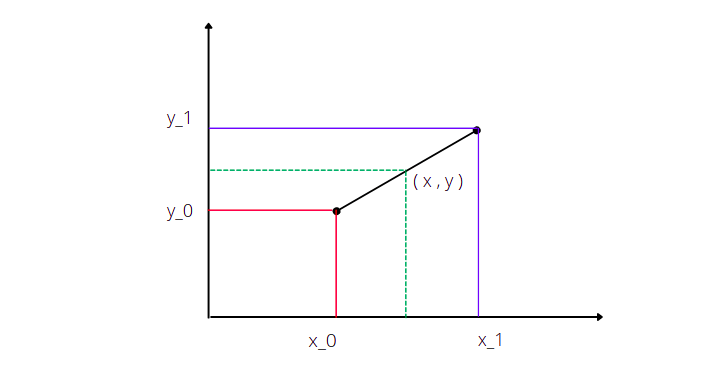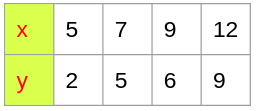Linear Interpolation

Linear interpolation creates continuous straight line for the data set by connecting the data points in the data set .It is a piece-wise function that is linear between every two adjacent data points.

By using this method we can easily find the corresponding values for the given value of ‘ x ‘ .

here we just use the two adjacent suitable values in which our desired value we need to calculate.$y =f(x)= y_1 + (y_2 - y_1)\frac {x-x_1}{x_2-x_1} , x_1 \leq x < x_2$ ,$y= f(x) = y_2 + (y_3 - y_2) \frac {x-x_2}{x_3-x_2} , x_2 \leq x < x_3$,

in general we can write that$y=f(x) = y_{n-1} + (y_n - y_{n-1}) \frac {x-x_{n-1}}{x_n-x_{n-1}} , x_{n-1} \leq x < x_n$Now let us try to find out the value of$y$  for$x = 10$

from the above equations$y= f(x) = 6 + (9 -6) \times \frac{10-9}{12-9} = 7$

hence by using linear interpolation the value obtained for$f(x) \ at \ x=10$ is$f(x) = 7$

Now let us try to find out the above values by using s2 !

				
%use s2
var datali = SortedOrderedPairs(
doubleArrayOf(5.0,7.0,9.0,12.0), //x-coordinates
doubleArrayOf(2.0,5.0,6.0,9.0)   //y-coordinates
)
var Li : LinearInterpolation = LinearInterpolation()
var f1: UnivariateRealFunction = Li.fit(datali)
//calculating the values of f(x) from corresponding x valyes
println(String.format("f(%f) = %f ", 6.0, f1.evaluate(6.0)))
println(String.format("f(%f) = %f ", 6.5, f1.evaluate(6.5)))
println(String.format("f(%f) = %f ", 8.0, f1.evaluate(8.0)))
println(String.format("f(%f) = %f ", 10.0, f1.evaluate(10.0)))
println(String.format("f(%f) = %f ", 11.0, f1.evaluate(11.0)))



Output :

f(6.000000) = 3.500000
f(6.500000) = 4.250000
f(8.000000) = 5.500000
f(10.000000) = 7.000000
f(11.000000) = 8.000000The draw backs of linear interpolation are
the curve we get is not smooth.The slopes experiecnes sudden changes because the first derivative of the neighbouring linear function does not agree at the point they meet. One of the most used method in practice to have a guarantee continuity of both first and second derivatives at the knots is Cubbic splines method

we will know much better about cubic splines below

Cubic Hermite Spline Interpolation

As the name Cubic Hermitre Spline Interpolation suggest that it is a peice wise spline interpolation where each peice is a third-degree polynomial in Hermite form ,that is determined by the values and first derivative at the two end points of the piece interval.
Now let us consider a single interval$[x_1 , x_2]$ given the values of$y_1$ at$x = x_1$ and similarly$y_2$ at$x = x_2$ with the tangents at$y_1 '$ at$x = x_1$ and$y_2 '$ at$x = x_2$,the segment curve can be defined by$f(x) = ax^3 + bx^2 + cx + d , x \in [ x_1 ,x_2]$

form the above statements we get the arbotory equations as$y_1 = ax_1^3 + bx_1^2 + cx_1 + d$$y_2 = ax_2^3 + bx_2^2 + cx_2 + d$$y_1 ' = 3ax_1^2 + 2bx_1 + c$$y_2 ' = 3ax_2^2 + 2bx_2 + c$
solving for the values a,b,c,d we get the interpolated curve on interval$[x_1 ,x_2]$
now let us try to find the value at a point bewteen the interval$[0,1]$
then here the values of$x_1 ,x-2$ becomes$x_1 = 0 , x_2 = 1$
upon susbtituting the values in the above arbitary equations and solving them for the values of a,b,c,d we get$f(x) = (2x^3-3x^2+1)y_1 + (x^3 -2x^2 +x)y_1 ' + (-2x^3 +3x^2)y_2 + (x^3-x^2)y_2 '$ where$x \in [0,1]$

we can substitute the values of x to find its corresponding value

for every point we need to find we have to use the adjacent boundaries that are given in the data set and to follow the same process as described above

it should be noted that the cubic hermite spline takes only values and tangents as inputs and no more .so that it ensures that the continuity of the first derivative but not the second derivative.

here when only data values are given as input ,the tangent values need to be estimated from them .
There are many methods that we use generally to calculate tangents but here in s2 there are 2 methods
they are

1. Catmull-Rom Spline Method
2. Finite-difference Method

Catmull-Rom Spline Method :

Now let us discuss more about Catmull-Rom Spline Method

we can calculate tangets by using the below method$y_k ' = \frac {y_{k+1} - y_{k-1}}{x_{k+1} - x_{k-1}}$
for the internal points$k = 2,...,n-1$ and$y_0 ' = \frac {y_1-y_0}{x_1-x_0} , \space y_n' = \frac{y_n - y_{n-1}}{x_n - x_{n-1}}$ at the end points

below explained the code for catmull-rom spline method

				
%use s2
var data_ch = SortedOrderedPairs(
doubleArrayOf(0.0, 0.7, 1.4, 2.1, 2.8, 3.5, 4.2, 4.9, 5.6, 6.3), //x-coordinates
doubleArrayOf(0.0, 0.644218, 0.98545, 0.863209, 0.334988, -0.350783,-0.871576, -0.982453, -0.631267, 0.0168139)   //y-coordinates
)
var ch : CubicHermite = CubicHermite(CubicHermite.Tangents.CATMULL_ROM)
var f_ch : UnivariateRealFunction =ch.fit(data_ch)
println(String.format("f(%f) = %f ", 2.0, f_ch.evaluate(2.0)))
println(String.format("f(%f) = %f ", 0.5, f_ch.evaluate(0.5)))
println(String.format("f(%f) = %f ", 1.7, f_ch.evaluate(1.7)))
println(String.format("f(%f) = %f ", 4.0, f_ch.evaluate(4.0)))
println(String.format("f(%f) = %f ", 6.0, f_ch.evaluate(6.0)))



Output :

f(2.000000) = 0.906031
f(0.500000) = 0.482239
f(1.700000) = 0.986796
f(4.000000) = -0.757465
f(6.000000) = -0.276516 

Finite difference :

finite difference estimates tangents as$y_k ' = \frac{1}{2} \times (\frac{y_{k+1} - y_k}{x_{k+1}-x_k} + \frac{y_k - y_{k-1}}{x_k - x_{k-1}} )$

for internal points k = 2,..,n-1 and one-sides difference$y_0 ' =\frac{y_1-y_0}{x_1 -x_0} , \space y_n '= \frac { y_n - y_{n-1}}{x_n - x_{n-1}}$ at the end points

				
%use s2
var data_ch = SortedOrderedPairs(
doubleArrayOf(0.0, 0.7, 1.4, 2.1, 2.8, 3.5, 4.2, 4.9, 5.6, 6.3), //x-coordinates
doubleArrayOf(0.0, 0.644218, 0.98545, 0.863209, 0.334988, -0.350783,-0.871576, -0.982453, -0.631267, 0.0168139)   //y-coordinates
)
var ch :CubicHermite=CubicHermite(CubicHermite.Tangents.FINITE_DIFFERENCE)
var f_ch : UnivariateRealFunction =ch.fit(data_ch)
println(String.format("f(%f) = %f ", 2.0, f_ch.evaluate(2.0)))
println(String.format("f(%f) = %f ", 0.5, f_ch.evaluate(0.5)))
println(String.format("f(%f) = %f ", 1.7, f_ch.evaluate(1.7)))
println(String.format("f(%f) = %f ", 4.0, f_ch.evaluate(4.0)))
println(String.format("f(%f) = %f ", 6.0, f_ch.evaluate(6.0)))



Output :

f(2.000000) = 0.906031
f(0.500000) = 0.482239
f(1.700000) = 0.986796
f(4.000000) = -0.757465
f(6.000000) = -0.276516 

Lorem ipsum dolor sit amet, consectetur adipiscing elit. Ut elit tellus, luctus nec ullamcorper mattis, pulvinar dapibus leo.